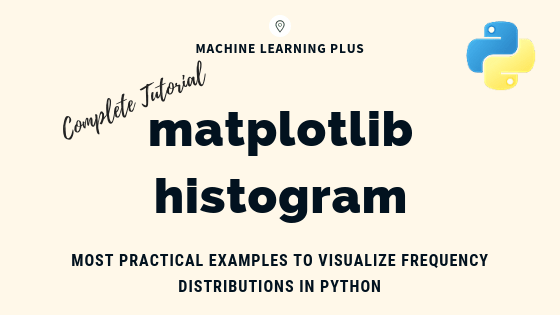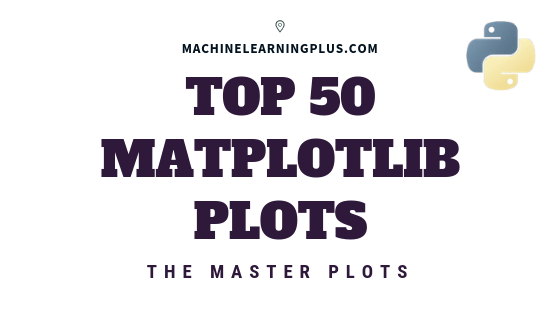Drop a Query

# Visualization## Matplotlib Histogram – How to Visualize Distributions in Python

Matplotlib histogram is used to visualize the frequency distribution of numeric array by splitting it to small equal-sized bins. In this article, we explore practical techniques that are extremely useful in your initial data analysis and plotting. Content What is a histogram? How to plot a basic histogram in python? Histogram grouped by categories in …## Matplotlib Tutorial – A Complete Guide to Python Plot with Examples

Until next time Until next time This tutorial explains matplotlib’s way of making plots in simplified parts so you gain the knowledge and a clear understanding of how to build and modify full featured matplotlib plots. 1. Introduction Matplotlib is the most popular plotting library in python. Using matplotlib, you can create pretty much any …## Top 50 matplotlib Visualizations – The Master Plots (with full python code)

A compilation of the Top 50 matplotlib plots most useful in data analysis and visualization. This list lets you choose what visualization to show for what situation using python’s matplotlib and seaborn library. Introduction The charts are grouped based on the 7 different purposes of your visualization objective. For example, if you want to picturize …Course Preview

## Machine Learning A-Z™: Hands-On Python & R In Data Science

### Free Sample Videos:#### Machine Learning A-Z™: Hands-On Python & R In Data Science#### Machine Learning A-Z™: Hands-On Python & R In Data Science#### Machine Learning A-Z™: Hands-On Python & R In Data Science#### Machine Learning A-Z™: Hands-On Python & R In Data Science